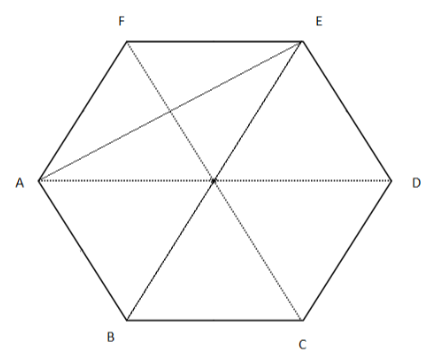Courses
Courses for Kids
Free study material
Free LIVE classes
MoreLIVE
Join Vedantu’s FREE Mastercalss

# If $ABCDEF$ is a regular hexagon. Find each interior angle of $\vartriangle ABE$ .Verified
364.2k+ views
Hint- To find out the angles of $\vartriangle ABE$, first determine each angle of hexagon by the help of general formula of net angle for regular polygon. Then find each equal angle and further proceed with the help of diagram.Given that $ABCDEF$ is a regular hexagon,
So all the sides will be equal in length.
Also we know that for $n -$ sided polygon.
Sum of all the interior angles $= (n - 2) \times {180^0}$.
Hence, sum of all the interior angles of hexagon is
$= (6 - 2) \times {180^0} \\ = 4 \times {180^0} \\ = {720^0} \\$
Also we know that for regular hexagon, all the interior angles are same so each angle
$= \dfrac{{{{720}^0}}}{6} \\ = {120^0}...........(1) \\$
Quadrilateral $ABEF$ is a $4$ sided polygon.
Sum of its interior angle
$= \left( {4 - 2} \right){180^0} \\ = 2 \times {180^0} \\ = {360^0} \\$
As $\angle AFE = {120^0}........(2)$ [from equation (1)]
In $\vartriangle AFE,$
$AF = EF.........(3)$ [Since they are sides of regular polygon]
$\Rightarrow \angle FAE = \angle FEA$ [Since angles opposite to equal sides are equal]
$\angle FAE + \angle FEA + \angle AFE = {180^0}$ [Sum of all the angle of triangle is ${180^0}$ ]
From equation (2) and equation (3) substituting the value
$\Rightarrow \angle FAE + \angle FEA + {120^0} = {180^0} \\ \Rightarrow \angle FAE + \angle FEA = {60^0} \\ \Rightarrow \angle FAE = \angle FEA = {30^0}[\because \angle FAE = \angle FEA] \\$
Since,$\angle FAE + \angle EAB = {120^0}$ [Angle of a regular hexagon]
$\therefore \angle EAB = {90^0}..........(4) \\ \angle ABE = \dfrac{1}{2} \times {120^0}\left[ {\because \angle ABE{\text{ bisects }}\angle ABC} \right] \\ = {60^0} \\$
Now moving on to triangle $ABE$
In $\vartriangle ABE$
$\angle AEB + \angle ABE + \angle EBA = {180^0}$ [Sum of all the angles of a triangle]
$\angle AEB = {180^0} - {60^0} - {90^0} = {30^0}$ [From equation (4) and equation (5)]
Hence all the angles of $\vartriangle ABE$ are
$\angle ABE = {60^0},\angle EAB = {90^0}\& \angle AEB = {30^0}$

Note- In order to solve such kind of questions of geometry, figure is a must in order to have a better understanding of the angles. Also all the general properties of triangles and polygons, like the sum of interior angles and relationship between the sides and angles, must be remembered. As, these properties are very handy in solving such problems.
Last updated date: 29th Sep 2023
Total views: 364.2k
Views today: 9.64k